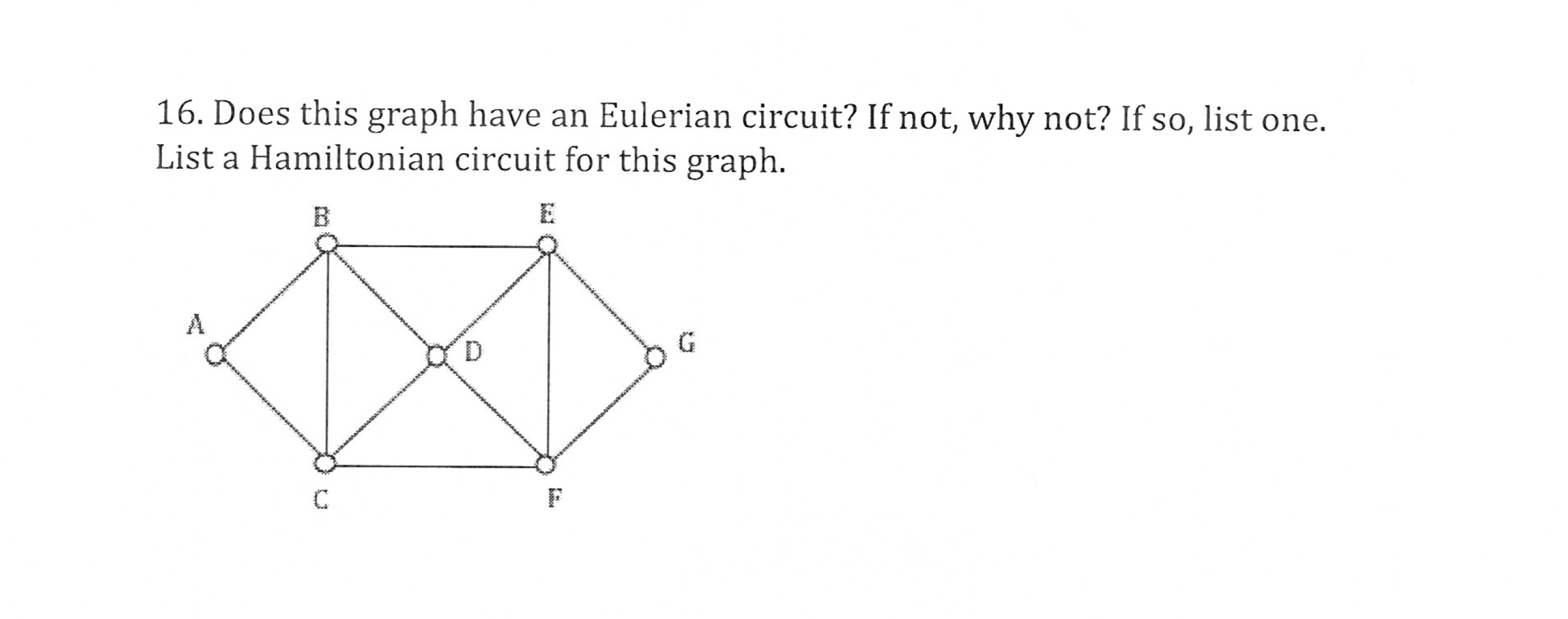# 16. Does this graph have an Eulerian circuit? If not, why not? If so, list one.List a Hamiltonian circuit for this graph

Question
15 viewshelp_outlineImage Transcriptionclose16. Does this graph have an Eulerian circuit? If not, why not? If so, list one. List a Hamiltonian circuit for this graph fullscreen
check_circle

Step 1

The given graph is shown in the below figure.

Step 2

Interpretation:

A graph is said to be Eulerian graph if it is a connected graph and every vertex of the graph has even degree.

Here, the degree of the vertex A, B, C, D, E, F and G are 2, 4, 4, 4, 4 and 2.

Since the graph is connected with all the vertices have even degree, the graph is an Eulerian.

Thus, Eulerian Circuit exits for the given graph.

Eulerian path is a path in graph that visits every edge exactly once. Eulerian Circuit is an Eulerian Path which starts and ends on the same vertex.

The Eulerian Circuit for the given graph is A-B-C-D-B-E-F-D-E-G-F-C-A.

Step 3

A Hamiltonian path is a path in a graph which visits each vertex exactly once. A Hamiltonian circuit is a cycle which visits each vertex exactly once and also returns to ...

### Want to see the full answer?

See Solution

#### Want to see this answer and more?

Solutions are written by subject experts who are available 24/7. Questions are typically answered within 1 hour.*

See Solution
*Response times may vary by subject and question.
Tagged in

### Math